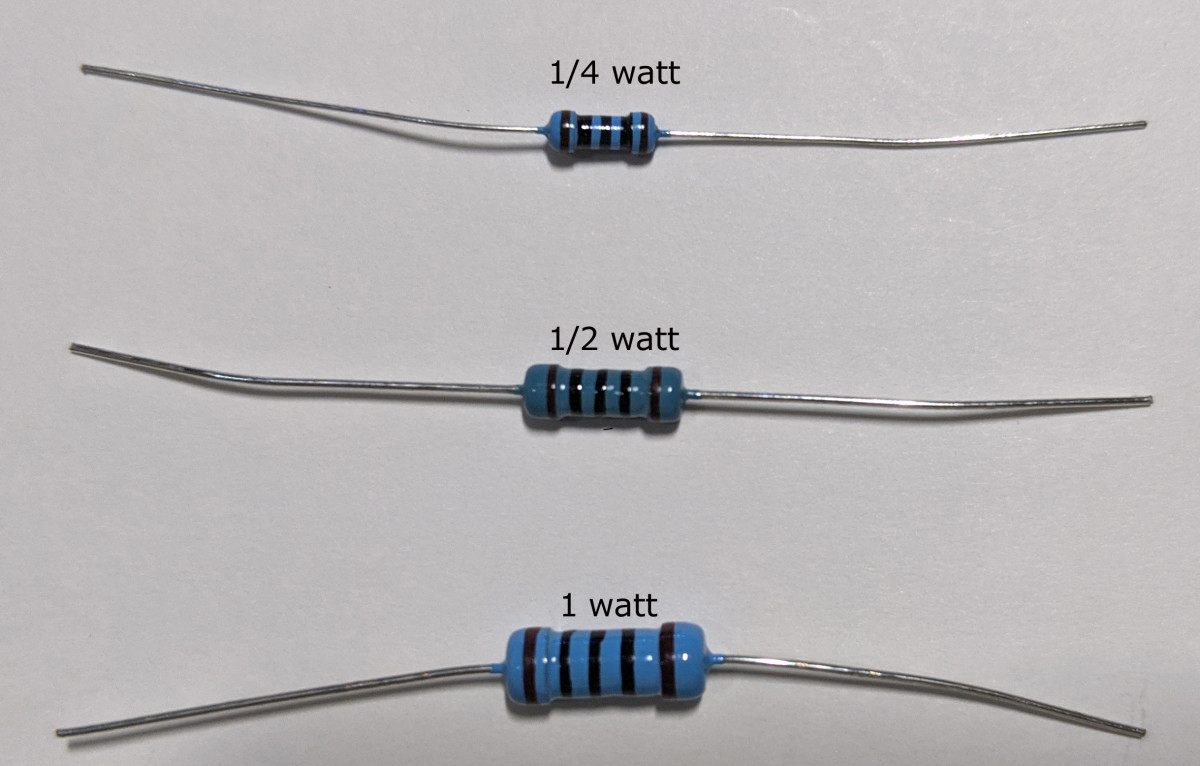# The Electronics Guy: Resistance Is (Not) Futile

• Author:
• Updated date:

## What Is A Resistor?

The job of a resistor is to constrict the flow of electricity.

There are many reasons for the need to restrict electrical flow, but the most common is to protect other components in a circuit from over-current.

Generally, an electronics circuit will only be supplied by a single voltage. The supply voltage will be that of whatever part of the circuit requires the highest voltage. However, such voltage may damage other components within the same circuit. Therefore, resistors are used to lower the voltage in those parts of the circuit and protect components from over-current caused by too much voltage. Since voltage is directly proportional to current but is inversely proportional to resistance. Meaning that a higher voltage will allow more current to flow, but a higher resistance will lower the current flow.

## Types of Resistors

Resistors are made of a semi-conductive material that lets a little bit of electricity through, but not all of it.

The most common types of resistors are carbon film, metal film, and ceramic. The type of material the resistor is made from is identified by the colour of the resistor itself. A light-brown resistor is made with carbon film, a green resistor is made from metal film, and ceramic resistors just look like rectangular blocks of ceramic material.

Resistors come in various power ratings, measured in watts. Although the wattage is not printed on the resistor, you can generally tell by the size. Ceramic resistors are usually only used for larger wattages like 5 or 10 watts. These are mostly used in industrial applications and are rarely seen in an electronics circuit since they are expensive, and such high power ratings are not required in low-voltage electronics. Most common are 1/4 watt and 1/2 watt metal film or carbon film. Less common, but available for use are 1/8 watt and 1 watt.

The reason for the different power ratings is that resistors produce energy in the form of heat while they are working. If a resistor gets too hot it will fail.

There is a mathematical formula used to calculate the required wattage, and it is recommended to use a higher wattage than required to extend the longevity of the resistor.

Scroll to ContinueResistors of the same resistance value (100 ohms) but of different wattages.

## Resistance Values

Of course, there are different resistance values as well. The amount of semi-conductive material used within the resistor determines its resistive value.

Resistance is measured in ohms using the Greek Omega symbol (Ω). Common values for resistors are, 10, 22, 47, 100, 150, 200, 220, 230, 470, 1k, 2k, 3k, 5k, 10k, 22k,47k,100k, 220k, 500k, and 1M.

Other resistance values can be achieved by connecting resistors together either in series or parallel. Connecting in series will increase the resistance. For example, two 230 Ω resistors in series will create a resistance of 460 Ω. Connecting resistors parallel to each other will decrease the resistance value. For example, two 10 Ω resistors in parallel will create a resistance of 5 Ω.

A mathematical formula called Ohm's Law is used to determine the required resistance. It is Electromagnetic Force (volts) equals Intensity (amps) multiplied by Resistance (ohms), E=IR. For example, if your circuit voltage is 5 volts, and you have a component that only needs 3.5 volts, you need to drop the voltage by 1.5. The said component draws 20 milliamps or 0.02 amps. That's 1.5 divided by 0.02, that is 75. Therefore, the required resistance is 75 ohms. Since there are no 75 ohn resistors, you would use the next closest value that is higher. Either two 47 ohms in series, or a single 100 ohm.

To calculate the wattage needed, you would use the Watt Formula. Power (watts) equals Intensity (amps) multiplied by Electromagnet Force (volts), P=IE. In that case, 1.5 times 0.02 equals 0.03 or 3/100 watts. Therefore a 1/4 watt or even a 1/8 watt resistor would be enough.

Resistance values are indicated on resistors by a series of coloured rings.

The below chart shows how to read resistance values.